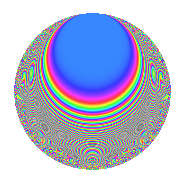Properties

 Label 109.2.fLevel 109 Weight 2 Character orbit f Rep. character $$\chi_{109}(16,\cdot)$$ Character field $$\Q(\zeta_{9})$$ Dimension 42 Newforms 1 Sturm bound 18 Trace bound 0

Related objects

Defining parameters

 Level: $$N$$ = $$109$$ Weight: $$k$$ = $$2$$ Character orbit: $$[\chi]$$ = 109.f (of order $$9$$ and degree $$6$$) Character conductor: $$\operatorname{cond}(\chi)$$ = $$109$$ Character field: $$\Q(\zeta_{9})$$ Newforms: $$1$$ Sturm bound: $$18$$ Trace bound: $$0$$

Dimensions

The following table gives the dimensions of various subspaces of $$M_{2}(109, [\chi])$$.

Total New Old
Modular forms 54 54 0
Cusp forms 42 42 0
Eisenstein series 12 12 0

Trace form

 $$42q$$ $$\mathstrut -\mathstrut 6q^{3}$$ $$\mathstrut -\mathstrut 12q^{4}$$ $$\mathstrut -\mathstrut 6q^{5}$$ $$\mathstrut +\mathstrut 12q^{6}$$ $$\mathstrut +\mathstrut 3q^{7}$$ $$\mathstrut -\mathstrut 12q^{8}$$ $$\mathstrut -\mathstrut 12q^{9}$$ $$\mathstrut +\mathstrut O(q^{10})$$ $$42q$$ $$\mathstrut -\mathstrut 6q^{3}$$ $$\mathstrut -\mathstrut 12q^{4}$$ $$\mathstrut -\mathstrut 6q^{5}$$ $$\mathstrut +\mathstrut 12q^{6}$$ $$\mathstrut +\mathstrut 3q^{7}$$ $$\mathstrut -\mathstrut 12q^{8}$$ $$\mathstrut -\mathstrut 12q^{9}$$ $$\mathstrut +\mathstrut 15q^{10}$$ $$\mathstrut -\mathstrut 15q^{11}$$ $$\mathstrut +\mathstrut 9q^{12}$$ $$\mathstrut -\mathstrut 30q^{13}$$ $$\mathstrut +\mathstrut 3q^{14}$$ $$\mathstrut +\mathstrut 6q^{16}$$ $$\mathstrut -\mathstrut 3q^{17}$$ $$\mathstrut -\mathstrut 27q^{18}$$ $$\mathstrut -\mathstrut 3q^{19}$$ $$\mathstrut -\mathstrut 30q^{20}$$ $$\mathstrut -\mathstrut 3q^{21}$$ $$\mathstrut -\mathstrut 18q^{22}$$ $$\mathstrut +\mathstrut 6q^{23}$$ $$\mathstrut -\mathstrut 12q^{24}$$ $$\mathstrut +\mathstrut 6q^{25}$$ $$\mathstrut +\mathstrut 15q^{26}$$ $$\mathstrut +\mathstrut 3q^{27}$$ $$\mathstrut +\mathstrut 66q^{28}$$ $$\mathstrut +\mathstrut 3q^{30}$$ $$\mathstrut +\mathstrut 6q^{31}$$ $$\mathstrut +\mathstrut 12q^{32}$$ $$\mathstrut +\mathstrut 24q^{33}$$ $$\mathstrut -\mathstrut 21q^{34}$$ $$\mathstrut -\mathstrut 54q^{35}$$ $$\mathstrut +\mathstrut 21q^{36}$$ $$\mathstrut -\mathstrut 24q^{37}$$ $$\mathstrut +\mathstrut 27q^{38}$$ $$\mathstrut +\mathstrut 18q^{39}$$ $$\mathstrut -\mathstrut 24q^{40}$$ $$\mathstrut -\mathstrut 30q^{41}$$ $$\mathstrut +\mathstrut 12q^{42}$$ $$\mathstrut +\mathstrut 9q^{43}$$ $$\mathstrut +\mathstrut 36q^{44}$$ $$\mathstrut +\mathstrut 12q^{45}$$ $$\mathstrut -\mathstrut 12q^{46}$$ $$\mathstrut -\mathstrut 42q^{47}$$ $$\mathstrut -\mathstrut 27q^{48}$$ $$\mathstrut +\mathstrut 15q^{49}$$ $$\mathstrut +\mathstrut 3q^{50}$$ $$\mathstrut -\mathstrut 12q^{51}$$ $$\mathstrut -\mathstrut 3q^{52}$$ $$\mathstrut +\mathstrut 3q^{53}$$ $$\mathstrut -\mathstrut 36q^{54}$$ $$\mathstrut +\mathstrut 21q^{55}$$ $$\mathstrut +\mathstrut 57q^{56}$$ $$\mathstrut -\mathstrut 15q^{57}$$ $$\mathstrut -\mathstrut 24q^{58}$$ $$\mathstrut +\mathstrut 18q^{59}$$ $$\mathstrut +\mathstrut 33q^{60}$$ $$\mathstrut +\mathstrut 6q^{61}$$ $$\mathstrut +\mathstrut 78q^{62}$$ $$\mathstrut -\mathstrut 48q^{63}$$ $$\mathstrut -\mathstrut 12q^{64}$$ $$\mathstrut +\mathstrut 3q^{65}$$ $$\mathstrut -\mathstrut 15q^{66}$$ $$\mathstrut -\mathstrut 6q^{67}$$ $$\mathstrut +\mathstrut 66q^{68}$$ $$\mathstrut +\mathstrut 15q^{69}$$ $$\mathstrut +\mathstrut 39q^{70}$$ $$\mathstrut +\mathstrut 15q^{71}$$ $$\mathstrut -\mathstrut 9q^{72}$$ $$\mathstrut +\mathstrut 66q^{73}$$ $$\mathstrut -\mathstrut 24q^{74}$$ $$\mathstrut +\mathstrut 24q^{75}$$ $$\mathstrut -\mathstrut 96q^{76}$$ $$\mathstrut -\mathstrut 39q^{77}$$ $$\mathstrut -\mathstrut 3q^{78}$$ $$\mathstrut +\mathstrut 18q^{79}$$ $$\mathstrut -\mathstrut 3q^{80}$$ $$\mathstrut -\mathstrut 15q^{81}$$ $$\mathstrut +\mathstrut 21q^{82}$$ $$\mathstrut +\mathstrut 21q^{83}$$ $$\mathstrut +\mathstrut 87q^{84}$$ $$\mathstrut +\mathstrut 120q^{85}$$ $$\mathstrut -\mathstrut 15q^{86}$$ $$\mathstrut +\mathstrut 12q^{87}$$ $$\mathstrut -\mathstrut 48q^{88}$$ $$\mathstrut +\mathstrut 15q^{89}$$ $$\mathstrut +\mathstrut 24q^{90}$$ $$\mathstrut +\mathstrut 63q^{92}$$ $$\mathstrut -\mathstrut 75q^{93}$$ $$\mathstrut -\mathstrut 30q^{94}$$ $$\mathstrut +\mathstrut 15q^{95}$$ $$\mathstrut -\mathstrut 21q^{96}$$ $$\mathstrut +\mathstrut 48q^{97}$$ $$\mathstrut -\mathstrut 126q^{98}$$ $$\mathstrut +\mathstrut 39q^{99}$$ $$\mathstrut +\mathstrut O(q^{100})$$

Decomposition of $$S_{2}^{\mathrm{new}}(109, [\chi])$$ into irreducible Hecke orbits

Label Dim. $$A$$ Field CM Traces $q$-expansion
$$a_2$$ $$a_3$$ $$a_5$$ $$a_7$$
109.2.f.a $$42$$ $$0.870$$ None $$0$$ $$-6$$ $$-6$$ $$3$$